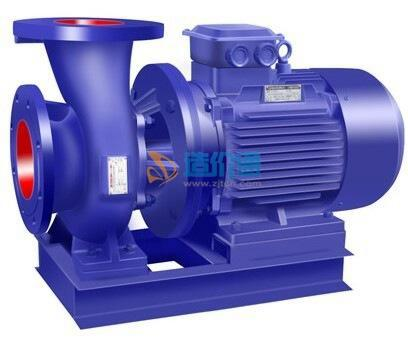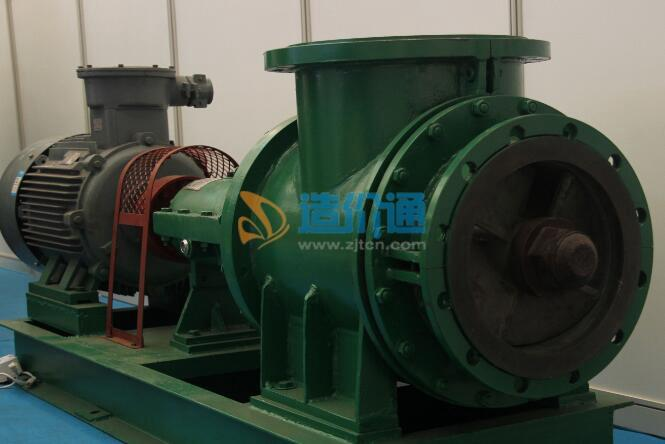[切换站点]

## 材料分类

Q=95m3/h,H=12m,N=5.5kw,铸铁• Q=95m3/h,H=12m,N=5.5kw,铸铁
13% 南京三元环保设备有限公司
2019-05

Q=25m3/h,H=10m,N=1.5kw,铸铁材质• Q=25m3/h,H=10m,N=1.5kw,铸铁材质

2019-05

Q=0.53m3/s H=3.1m P=30kw• Q=0.53m3/s H=3.1m P=30kw
13% 南京兰江泵业有限公司
2019-05

Q=1.65m3/s H=2.9m P=110kw• Q=1.65m3/s H=2.9m P=110kw
13% 南京兰江泵业有限公司
2019-05

Q=2.3m3/s H=5.5m P=185kw• Q=2.3m3/s H=5.5m P=185kw
13% 南京兰江泵业有限公司
2019-05

Q=2.65m3/s H=5.2m P=220kw• Q=2.65m3/s H=5.2m P=220kw
13% 南京兰江泵业有限公司
2019-05

Q=5.5m3/s H=6.5m P=500kw• Q=5.5m3/s H=6.5m P=500kw
13% 南京兰江泵业有限公司
2019-05

2AP• 2AP
13% 南京中环环保设备有限公司
2019-05

2AP• 2AP
13% 南京兰江泵业有限公司
2019-05

Q=95m3/h,H=12m,N=5.5kw,铸铁材质• Q=95m3/h,H=12m,N=5.5kw,铸铁材质

2019-05

AS55-4CB 流量 100m3/h 扬程 7.5mH2O• AS55-4CB 流量 100m3/h 扬程 7.5mH2O

2019-05

2AP• 2AP
13% 南京维克环保设备有限公司
2019-05

50WQ10-10-0.75 Q=10m3／h,H=10m,P=0.75KW• 50WQ10-10-0.75 Q=10m3／h,H=10m,P=0.75KW
13% 昆山三艾斯工业用品有限公司
2019-05

CP50.75-50 Q=10 m3/h,H=10m,P=0.75kW• CP50.75-50 Q=10 m3/h,H=10m,P=0.75kW

2019-05

Q=30m3 /h H=15m N=4KW 65XWQ-30-15-4.0• Q=30m3 /h H=15m N=4KW 65XWQ-30-15-4.0
13% 宜兴市概念环保设备有限公司
2019-05

ZQB、HQB型潜水轴流泵、混流泵
ZQB/HQB/ZQB.HQB 不锈钢ZQB、HQB型潜水轴流泵、混流泵（轴流泵）
• ZQB/HQB/ZQB.HQB 不锈钢

2019-05